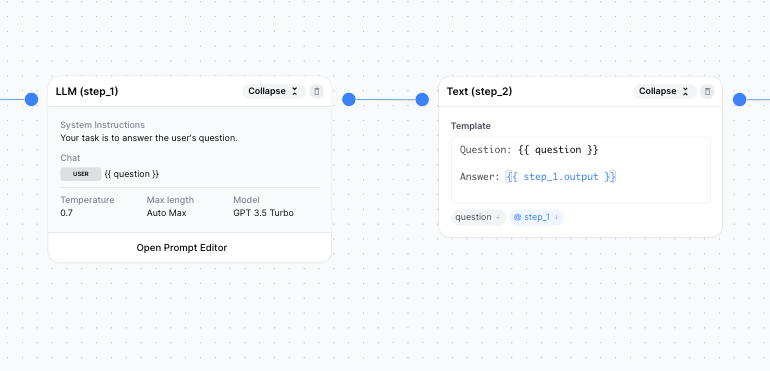# How do I reference outputs?

Just like with inputs, you can reference the outputs of previous steps in any subsequent step.

Currently, step names are automatically defined: step_1, step_2, so on and so forth. To reference the output of a step, simply type {{ step_1.output }} (where 1 is the number of the step)."What is the output exactly?" you might ask. Great question! In AirOps, you can view the exact output of a step in the Test panel (note: you can only view the output of the final step in a workflow).

In our example, "what should I name my corgi?", {{ step_1.output }} would be the result from GPT as shown here: